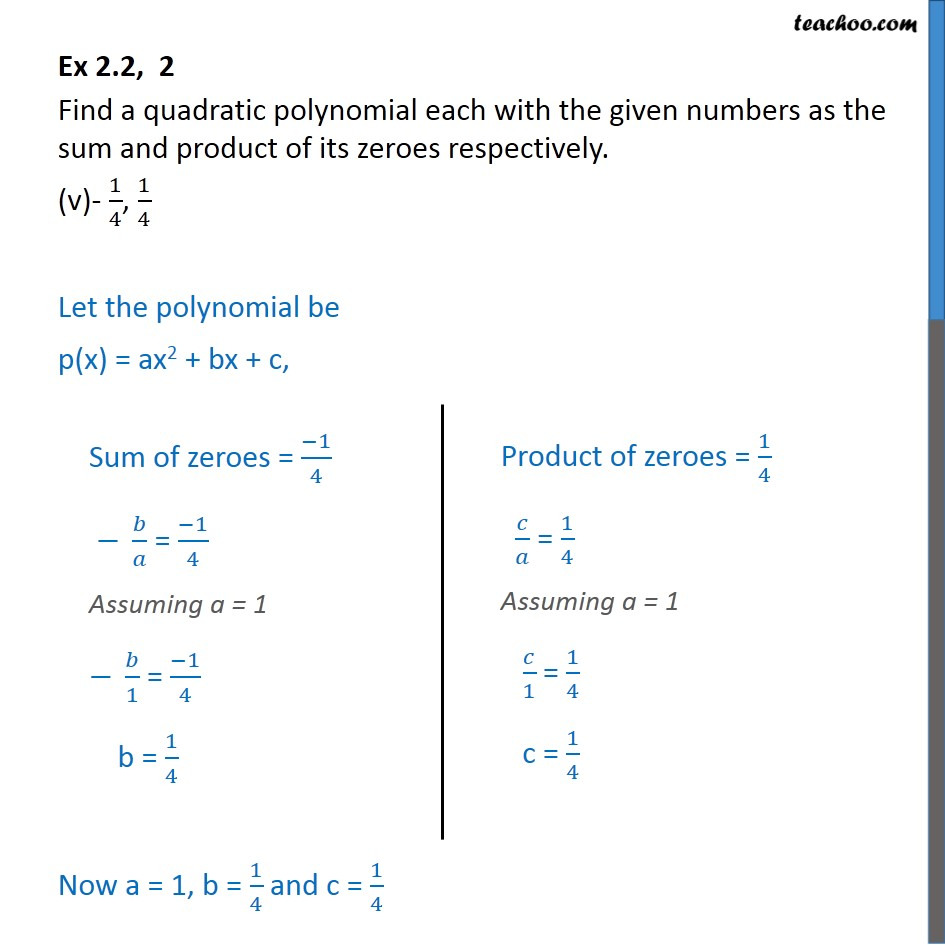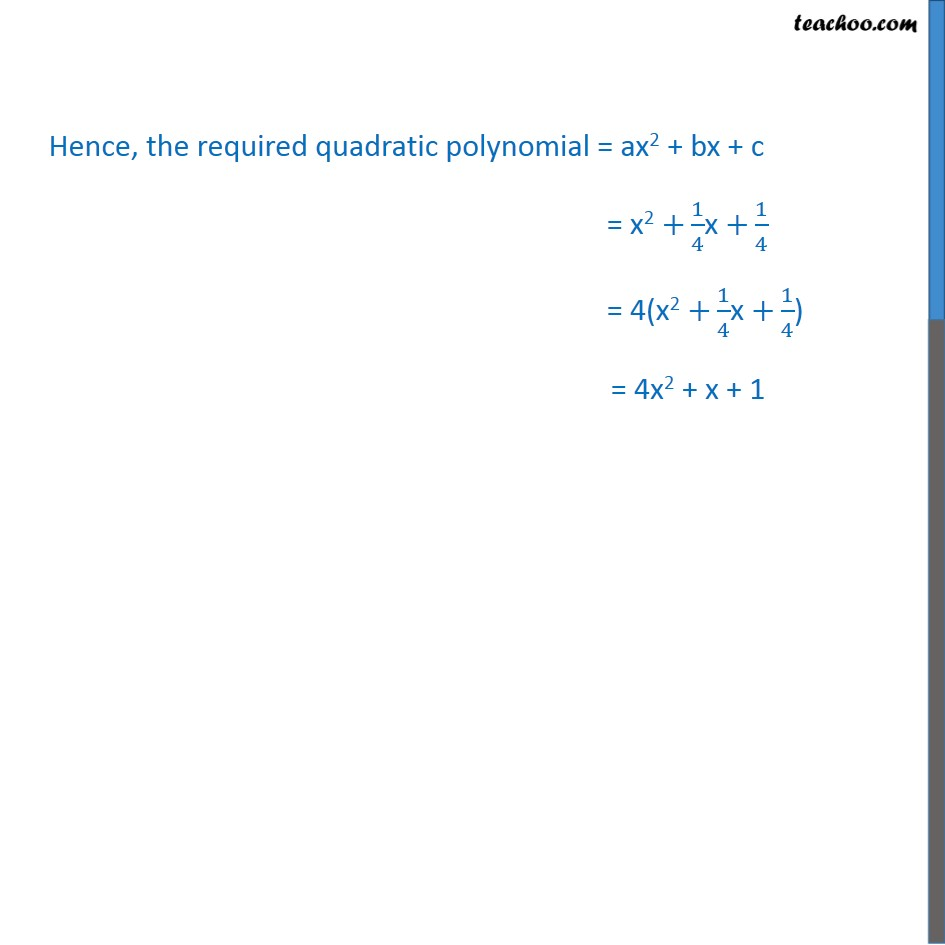1. Chapter 2 Class 10 Polynomials (Term 1)
2. Serial order wise
3. Ex 2.2

Transcript

Ex 2.2, 2 Find a quadratic polynomial each with the given numbers as the sum and product of its zeroes respectively. (v)- 1/4, 1/4 Let the polynomial be p(x) = ax2 + bx + c, Now a = 1, b = 1/4 and c = 1/4 Hence, the required quadratic polynomial = ax2 + bx + c = x2 +1/4x +1/4 = 4(x2 +1/4x +1/4)

Ex 2.2